Limits - Limit exists

Chapter 13 Class 11 Limits and Derivatives (Term 1 and Term 2)
Concept wise

For limits,

we put value and check if it is of the form 0/0, ∞/∞, 1

If it is of that form, we cannot find limits by putting values.

We use limit formula to solve it.

We have provided all formulas of limits like

## Limits of Trigonometry Functions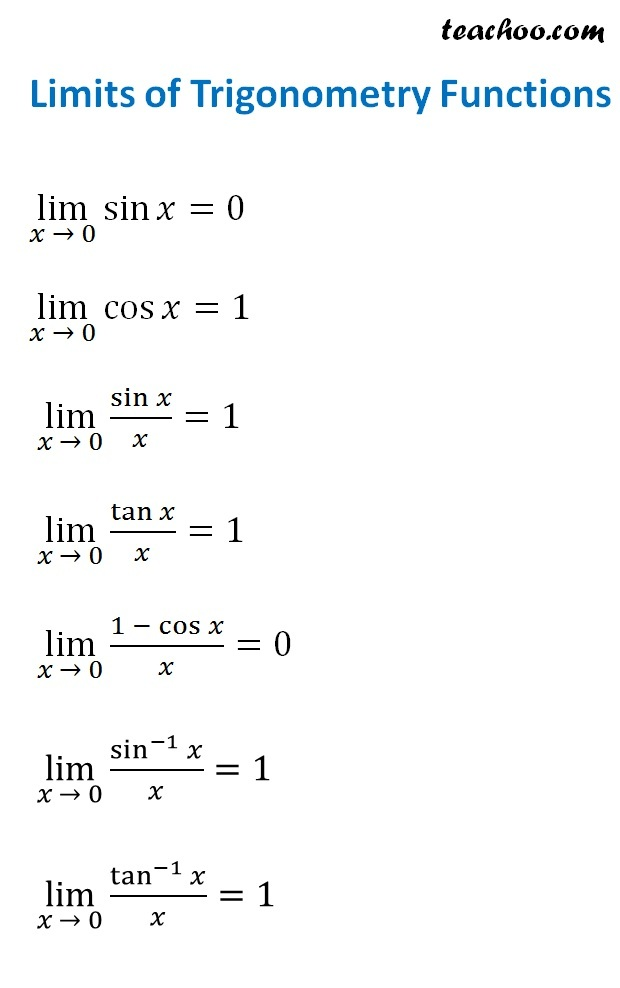## Limits of Log and Exponential Functions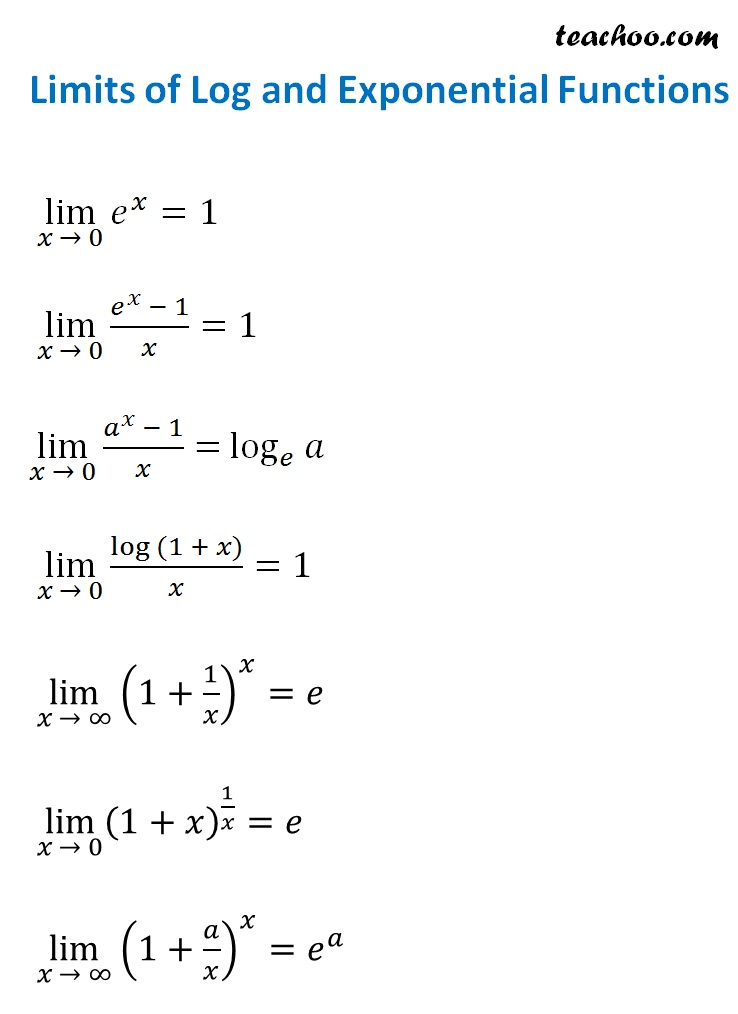## Limits of the form 1 ∞ and x^n formula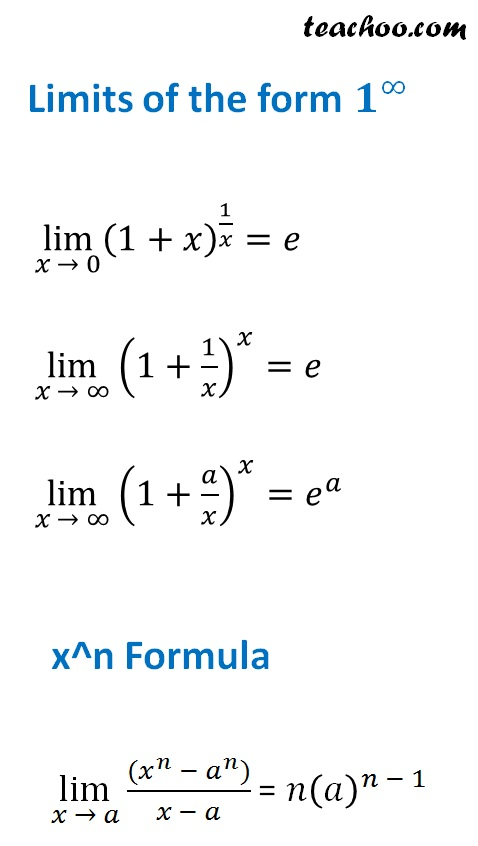## Checking if Limit Exists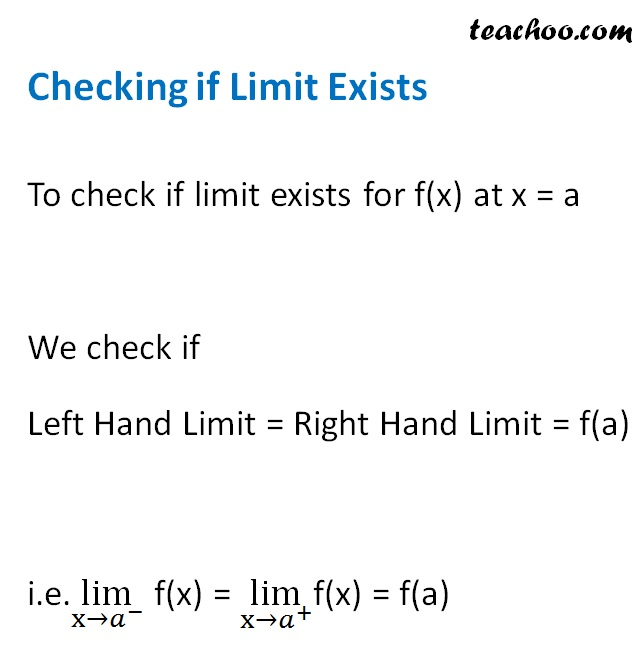## L'hospital’s rule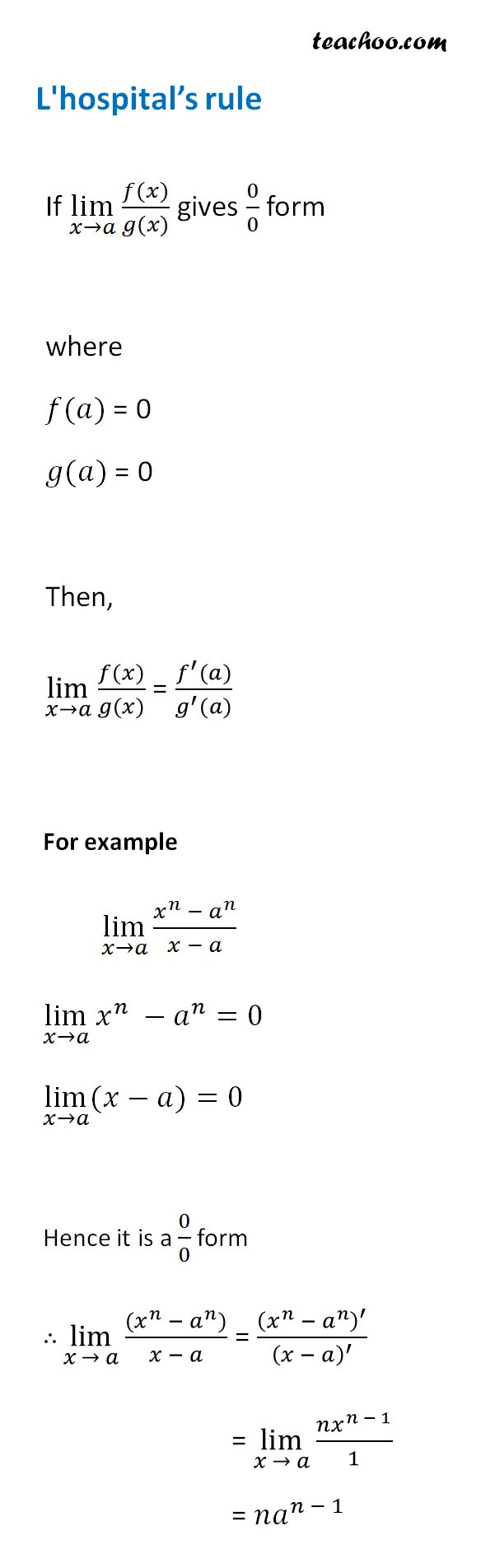### Transcript

Limits of Trigonometry Functions lim┬(𝑥 → 0)⁡sin⁡𝑥 =0 lim┬(𝑥 → 0)⁡cos⁡𝑥 =1 lim┬(𝑥 → 0)⁡〖sin⁡𝑥/𝑥〗=1 lim┬(𝑥 → 0)⁡〖tan⁡𝑥/𝑥〗=1 lim┬(𝑥 → 0)⁡〖(1 − cos⁡𝑥)/𝑥〗=0 lim┬(𝑥 → 0)⁡〖sin^(−1)⁡𝑥/𝑥〗=1 lim┬(𝑥 → 0)⁡〖tan^(−1)⁡𝑥/𝑥〗=1 Limits of Log and Exponential Functions lim┬(𝑥 → 0)⁡〖𝑒^𝑥 〗=1 lim┬(𝑥 → 0)⁡〖(𝑒^𝑥 − 1)/𝑥〗=1 lim┬(𝑥 → 0)⁡〖(𝑎^𝑥 − 1)/𝑥〗= log_𝑒⁡𝑎 lim┬(𝑥 → 0)⁡〖〖log 〗⁡〖(1 + 𝑥)〗/𝑥〗=1 lim┬(𝑥 → ∞)⁡〖(1+1/𝑥)^𝑥 〗=𝑒 lim┬(𝑥 → 0)⁡〖(1+𝑥)^(1/𝑥) 〗=𝑒 lim┬(𝑥 → ∞)⁡〖(1+𝑎/𝑥)^𝑥 〗=𝑒^𝑎 Limits of the form 𝟏^∞ lim┬(𝑥 → 0)⁡〖(1+𝑥)^(1/𝑥) 〗=𝑒 lim┬(𝑥 → ∞)⁡〖(1+1/𝑥)^𝑥 〗=𝑒 lim┬(𝑥 → ∞)⁡〖(1+𝑎/𝑥)^𝑥 〗=𝑒^𝑎 x^n Formula lim┬(𝑥 → 𝑎)⁡〖((𝑥^𝑛 − 𝑎^𝑛))/(𝑥 − 𝑎)〗 = 𝑛(𝑎)^(𝑛 − 1) To check if limit exists for f(x) at x = a We check if Left Hand Limit = Right Hand Limit = f(a) i.e.lim┬(〖x→𝑎〗^− ) f(x) = lim┬(〖x→𝑎〗^+ )f(x) = f(a) L'hospital’s rule If lim┬(𝑥→𝑎)⁡〖(𝑓(𝑥))/(𝑔(𝑥))〗 gives 0/0 form where 𝑓(𝑎) = 0 𝑔(𝑎) = 0 Then, lim┬(𝑥→𝑎)⁡〖(𝑓(𝑥))/(𝑔(𝑥))〗 = (𝑓^′ (𝑎))/(𝑔^′ (𝑎)) For example lim┬(𝑥→𝑎)⁡〖(𝑥^𝑛 − 𝑎^𝑛)/(𝑥 − 𝑎)〗 lim┬(𝑥→𝑎)⁡〖𝑥^𝑛 −𝑎^𝑛 〗=0 lim┬(𝑥→𝑎)⁡〖(𝑥−𝑎)〗=0 Hence it is a 0/0 form ∴ lim┬(𝑥 → 𝑎)⁡〖((𝑥^𝑛 − 𝑎^𝑛))/(𝑥 − 𝑎)〗 = (𝑥^𝑛 − 𝑎^𝑛 )^′/(𝑥 − 𝑎)^′ = lim┬(𝑥 → 𝑎)⁡〖(𝑛𝑥^(𝑛 − 1))/1〗 = 𝑛𝑎^(𝑛 − 1)